# Hex, Bugs and More Physics | Emre S. Tasci

a blog about physics, computation, computational physics and materials…

# How to Prepare an Input File for Surface Calculations

## Emre S. Tasci

19/07/2013

Abstract

This how-to is intended to guide the reader during the preparation of the input file for an intended surface calculation study. Mainly VESTA software is used to manipulate and transform initial structure file along with some home brewed scripts to do simple operations such as translations.

# Description

One of the questions I’m frequently being asked by the students is the preparation of input files for surface calculations (or more accurately, “a practical way” for the purpose). In this text, starting from a structural data file for bulk, the methods to obtain the input file fit for structure calculations will be dealt.

# Tools

VESTA [A] is a free 3D visualization program for structural models that also allows editing to some extent. It is available for Windows, MacOS and Linux operating systems.
STRCONVERT [B] is a tool hosted on the Bilbao Crystallographic Server[] (BCS) that offers visualization and also basic editing as well as conversion between various common structural data formats.
TRANSTRU [C] is an another BCS tool that transforms a given structure via the designated transformation matrix.

# Procedure

## Initial structural data

We start by obtaining the structural data for the unit cell of the material we are interested in. It should contain the unit cell dimensions and the atomic positions. Some common formats in use are: CIF[], VASP[], VESTA[] and BCS formats. Throughout this text, we will be operating on the Fm3mphase of the platinum carbide (PtC), reported by Zaoui & Ferhat[] as reproduced in BCS format in Table 1↓.

225
4.50 4.50 4.50 90. 90. 90.
2
C 1 4a 0 0 0
Pt 1 4b 0.5 0.5 0.5

Table 1 Structural data of Fm3m PtC
These data can be entered directly into VESTA from the form accessed by selecting the “File→New Structure” menu items and then filling the necessary information into the “Unit cell” and “Structure parameters” tabs, as shown in Figures 1↓ and 2↓.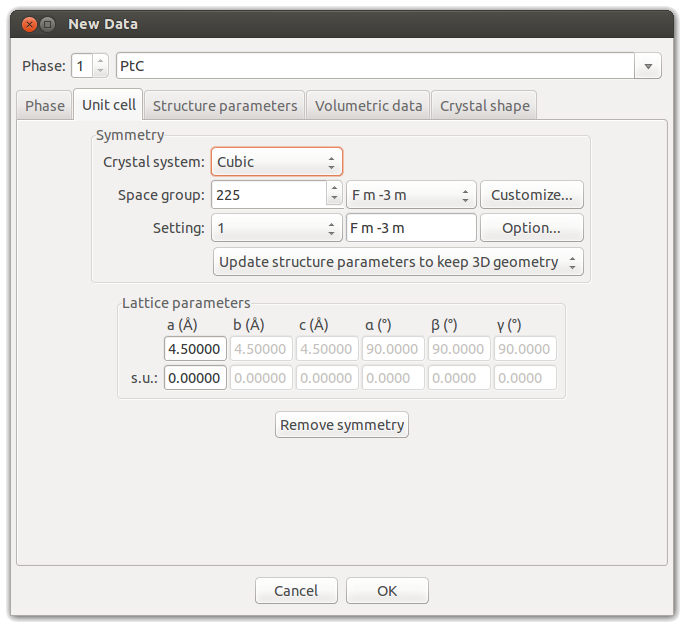Figure 1 VESTA’s “Unit cell” interface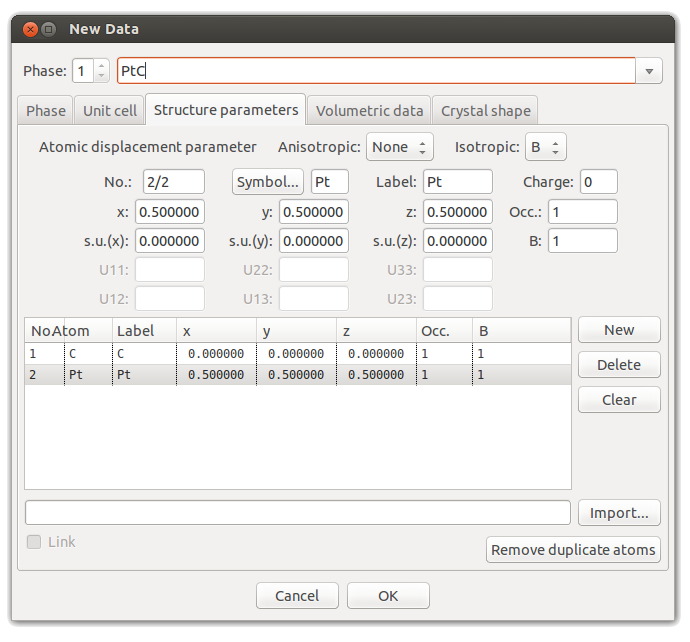Figure 2 VESTA’s “Structure parameters” interface
As a result, we have now defined the Fm3m PtC (Figure 3↓).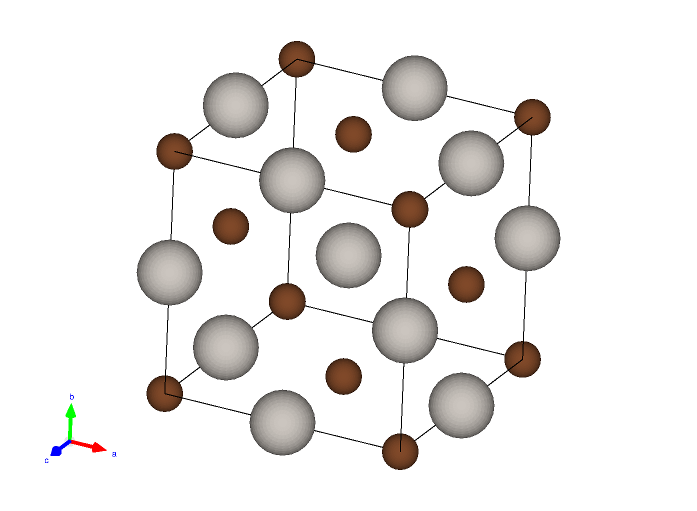Figure 3 Fm3m platinum carbide
An alternative way to introduce the structure would be to directly open its structural data file (obtained from various structure databases such as COD[], ICSD[] or Landolt-Bornstein[] into VESTA.

## Constructing the Supercell

A supercell is a collection of unit cells extending in the a,b,c directions. It can easily be constructed using VESTA’s transformation tool. For demonstration purposes, let’s build a 3x3x3 supercell from our cubic PtC cell. Open the “Edit→Edit Data→Unit Cell…” dialog, then click the “Option…” button in the “Setting” row. Then define the transformation matrix as “3a,3b,3c” as shown in Figure 4↓.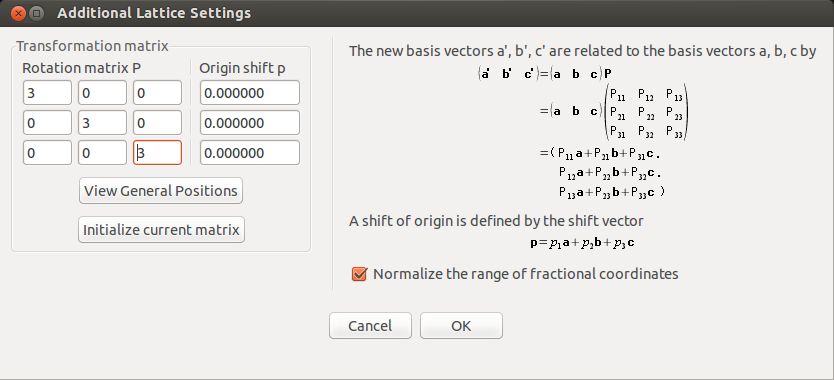Figure 4 Transformation matrix for the supercell
Answer “yes” when the warning for the change of the volume of the unit cell appears, and again click “Yes” to search for additional atoms. After this, click “OK” to exit the dialog. This way we have constructed a supercell of 3x3x3 unit cells as shown in Figure 5↓.Figure 5 A 3x3x3 supercell

,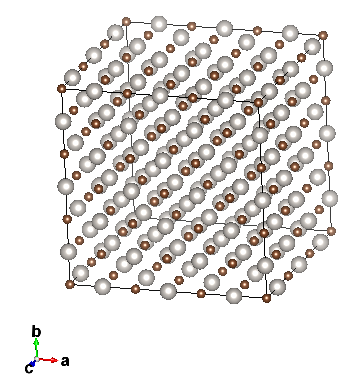Figure 6 Designating the boundaries for the construction of the supercell.
We have built the supercell for a general task but at this stage, it’s not convenient for preparing the surface since we first need to cleave with respect to the necessary lattice plane. So, revert to the conventional unit cell (either by reopening the data file or undoing (CTRL-z) our last action).
Now, populate the space with the conventional cell using the “Boundary” setting under the “Style” tool palette (Figure 6↑). In this case, we are building a 3x3x3 supercell by including the atoms within the -fractional- coordinate range of [0,3] along all the 3 directions (Figure 7↓).Figure 7 (a)Single unit cell (conventional); (b) 3x3x3 supercell
At this stage, the supercell formed is just a mode of display – we haven’t made any solid changes yet. Also, if preferred one can choose how the unit cells will be displayed from the “Properties” setting’s “General” tab.

## Lattice Planes

To visualize a given plane with respect to the designated hkl values, open the dialog shown in Figure 8↓ by selecting “Edit→Lattice Planes…” from the menu, then clicking on “New” and entering the intended hkl values, in our example (111) plane.

etasci@metu.edu.tr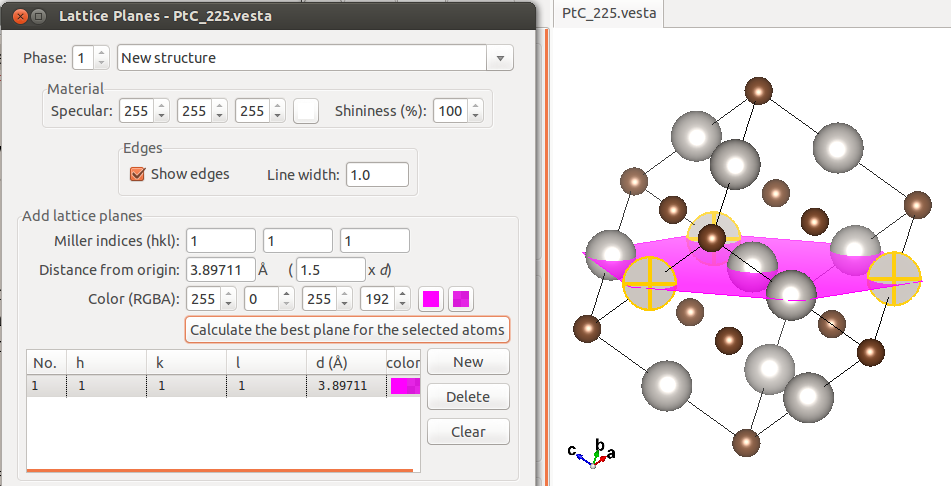Figure 8 Selecting the (111) lattice plane
The plane’s distance to the origin can be specified in terms of Å or interplane distance, as well as selecting 3 or more atoms lying on the plane (multiple selections can be realised by holding down SHIFT while clicking on the atoms) and then clicking on the “Calculate the best plane for the selected atoms” button to have the plane passing from those positions to appear. This is shown in Figure 9↓, done within a 3x3x3 supercell.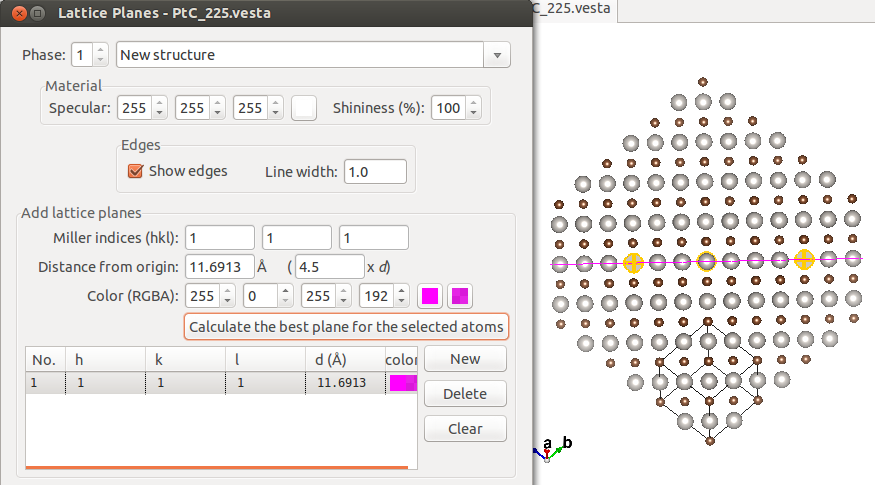Figure 9 Selecting the (111) lattice plane in the 3x3x3 supercell
The reason we have oriented our system so that the normal to the (111) plane is pointing up is to designate this lattice plane as the surface, aligning it with the new c axis for convenience. Therefore we also need to reassign a and b axes, as well. This all comes down to defining a new unit cell. Preserving the periodicities, the new unit cell is traced out by introducing additional lattice planes. These new unit cells are not unique, as long as one preserves the periodicities in the 3 axial directions, they can be taken as large and in any convenient shape as allowed. A rectangular shaped such a unit cell, along with the outlying lattice plane parameters is presented in Figure 10↓ (the boundaries have been increased to [-2,5] in each direction for practicality).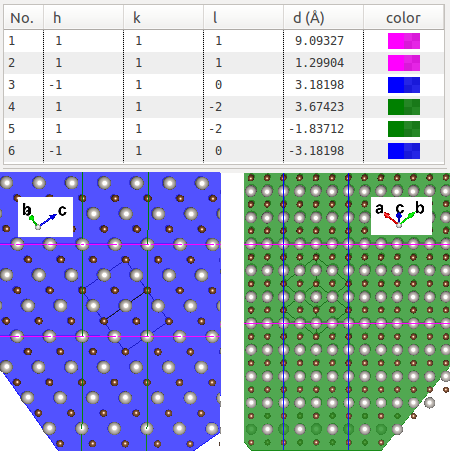Figure 10 The new unit cell marked out by the lattice plane “boundaries”

## Trimming the new unit cell

The next procedure is simple and direct: remove all the atoms outside the new unit cell. You can use the “Select” tool from the icons on the left (the 2nd from top) or the shortcut key “s”. The “trimmed” new unit cell is shown in Figure 11↓, take note of the periodicity in each section. It should be noted that, this procedure is only for deducing the transformation matrix, which we’ll be deriving in the next subsection: other than that, since it’s still defined by the “old” lattice vectors, it’s not stackable to yield the correct periodicity so we’ll be fixing that.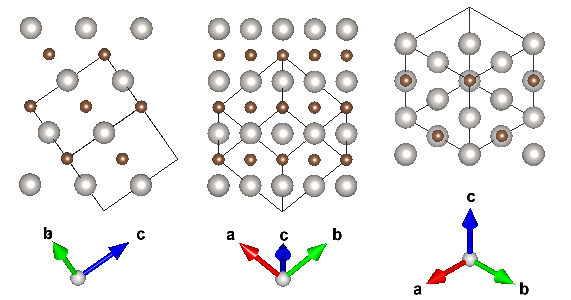Figure 11 Filtered new unit cell with the original cell’s lattice vectors

## Transforming into the new unit cell

Now that we have the new unit cell, it is time to transform the unit cell vectors to comply with the new unit cell. For the purpose of obtaining the transformation matrix (which actually is the matrix that relates the new ones with the old ones), we will need the coordinates of the 4 atoms on the edges. Such a set of 4 selected atoms are shown in Figure 12↓. These atoms’ fractional coordinates are as listed in Table 2↓ (upon selecting an atom, VESTA displays its coordinates in the information panel below the visualization).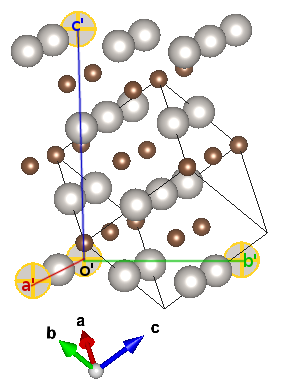Figure 12 The reference points for the new axe1s

 Label a’ b’ c’ o’ a 0 1/2 2 1 b 1 -1/2 1 0 c -1/2 1/2 1/2 -1/2
Table 2 Reference atoms’ fractional coordinates
Taking o’ as our new origin, the new lattice vectors in terms of the previous ones become (as we subtract the “origin’s” coordinates):
a’ = -a+b
b’ = -1/2a-1/2b+c
c’ = a+b+c
Therefore our transformation matrix is:
− 1 − 121 1 − 121 011
(i.e., “-a+b,-1/2a-1/2b+c,a+b+c” — the coefficients are read in columns)
Transforming the initial cell with respect to this matrix is pretty straight forward, in the same manner we exercised in subsection 3.2↑, “Constructing the supercell”, i.e., “Edit→Edit Data→Unit Cell..”, then “Remove symmetry” and “Option…” and introducing the transformation matrix. A further translation (origin shift) of (0,0,1/2) is necessary (the transformation matrix being “-a+b,-1/2a-1/2b+c,a+b+c+1/2”) if you want the transformed structure look exactly like the one shown in Figure 11↑.

### A Word of caution

Due to a bug, present in VESTA, you might end with a transformed matrix with missing atoms (as shown in Figure – compare it to the middle and rightmost segments of Figure 11↑). For this reason, always make sure you check your resulting unit cell thoroughly! (This bug has recently been fixed and will be patched in the next version (3.1.7)-personal communication with K.Momma)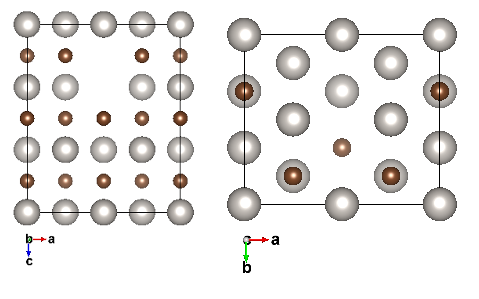Figure 13 The transformed structure with missing atoms
To overcome this problem, one can conduct the transformation via the TRANSTRU [E] tool of the Bilbao Crystallographic Server. Enter your structural data (either as a CIF or by definition into the text box), select “Transform structure to a subgroup basis”, in the next window enter “1” as the “Low symmetry Space Group ITA number” (1 corresponding to the P1 symmetry group) and the transformation matrix either in abc notation (“-a+b,-1/2a-1/2b+c,a+b+c+1/2”) or by directly entering the matrix form (both are filled in the screenshot taken in Figure 14↓).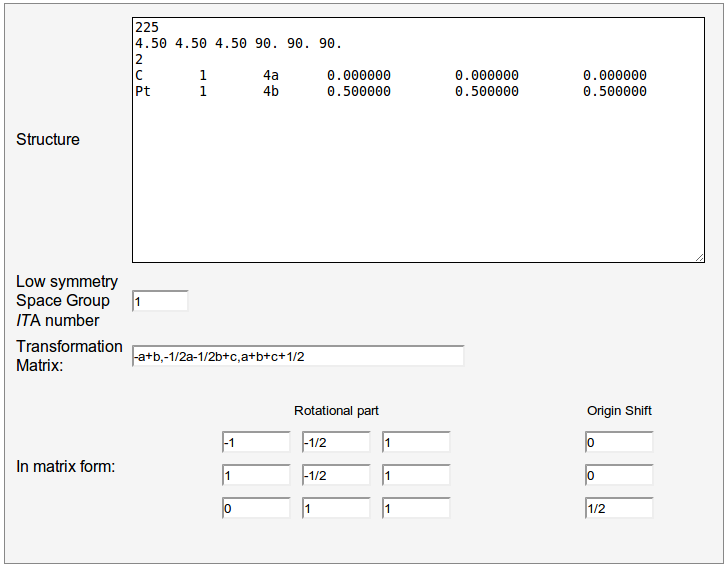Figure 14 TRANSTRU input form
In the result page export the transformed structure data (“Low symmetry structure data”) to CIF format by clicking on the corresponding button and open it in VESTA. This way all the atoms in the new unit cell will have been included.

## Final Touch

The last thing remains is introducing a vacuum area above the surface. For this purpose we switch from fractional coordinates to Cartesian coordinates, so when the cell boundaries are altered, the atomic positions will not change as a side result. VASP format supports both notations so we export our structural data into VASP format (“File→Export Data…”, select “VASP” as the file format, then opt for “Write atomic coordinates as: Cartesian coordinates”).
After the VASP file with the atomic coordinates written in Cartesian format is formed, open it with an editor (the contents are displayed in Table3↓).

generated_by_bilbao_crystallographic_server

1.0

6.3639612198 0.0000000000 0.0000000000

0.0000000000 5.5113520622 0.0000000000

0.0000000000 0.0000000000 7.7942290306

C Pt

12 12

Cartesian

0.000000000 3.674253214 6.495164688

0.000000000 1.837099013 1.299064110

3.181980610 1.837099013 1.299064110

1.590990305 0.918577018 6.495164688

4.772970915 4.592774880 1.299064110

1.590990305 4.592774880 1.299064110

4.772970915 0.918577018 6.495164688

0.000000000 0.000000000 3.897114515

3.181980610 0.000000000 3.897114515

4.772970915 2.755676031 3.897114515

1.590990305 2.755676031 3.897114515

3.181980610 3.674253214 6.495164688

0.000000000 3.674253214 2.598050405

1.590990305 0.918577018 2.598050405

4.772970915 0.918577018 2.598050405

1.590990305 4.592774880 5.196178858

3.181980610 1.837099013 5.196178858

0.000000000 1.837099013 5.196178858

4.772970915 4.592774880 5.196178858

0.000000000 0.000000000 0.000000000

3.181980610 3.674253214 2.598050405

3.181980610 0.000000000 0.000000000

4.772970915 2.755676031 0.000000000

1.590990305 2.755676031 0.000000000
Table 3 The constructed (111) oriented VASP file’s contents
Directly edit and increase the c-lattice length from the given value (7.7942 Å in our case) to a sufficiently high value (e.g., 25.0000 Å) and save it. Now when you open it back in VESTA, the surface with its vacuum should be there as in Figure 15↓.Figure 15 Prepared surface with vacuum
At this point, there might be a couple of questions that come to mind: What are those Pt atoms doing at the top of the unit cell? Why are the C atoms positioned above the batch instead of the Pt atoms?
The answer to these kind of questions is simple: Periodicity. By tiling the unit cell in the c-direction using the “Boundary…” option and designating our range of coordinates for the {x,y,z}-directions from 0 to 3, we obtain the system shown in Figure 16↓.Figure 16 New unit cell with periodicity applied
From the periodicity, in fractional coordinates, f|z = 0 = f|z = 1, meaning the atoms at the base and at the very top of the unit cell are the same (or “equivalent” from the interpretational side). If we had wanted the Pt atoms to be the ones forming the surface, then we would have made the transformation with the “-a+b,-1/2a-1/2b+c,a+b+c” matrix instead of “-a+b,-1/2a-1/2b+c,a+b+c+1/2”, so the half shift would amount the change in the order, and then increase the c-lattice parameter size in the Cartesian coordinates notation (i.e., in the VASP file). The result of such a transformation is given in Figure 17↓.Figure 17 Alternative surface with the Pt atoms on the top (Left: prior to vacuum introduction; Center: with vacuum; Right: tiled in periodicity)

# Alternative Ways

In this work, a complete treatment of preparing a surface in silico is proposed. There are surely more direct and automated tools that achieve the same task. It has been brought to our attention that the commercial software package Accelrys Materials Studio [F] has an integrated tool called “Cleave Surface” which exactly serves for the same purpose discussed in this work. Since it is propriety software, it will not be further described here and we encourage the user to benefit from freely accessible software whenever possible.
Also, it is always to compare your resulting surface with that of an alternate one obtained using another tool. In this sense, Surface Explorer [G] is very useful in visualizing how the surface will look, eventhough its export capabilities are limited.
Chapter 4 (“DFT Calculations for Solid Surfaces”) of Zhang’s Ph.D. thesis[] contains very fruitful discussions on possible ways to incorporate the surfaces in computations.
It is the author’s intention to soon implement such an automated tool for constructing surfaces from bulk data, integrated within the Bilbao Crystallographic Server’s framework of tools.

# Conclusion

Preparing a surface fit for atomic & molecular calculations can be tedious and tiresome. In this work, we have tried to guide the reader in a step-by-step procedure, sometimes wandering off from the direct path to show the mechanisms and reasons behind the usually taken on an “as-it-is” basis, without being pondered upon. This way, we hope that the techniques acquired throughout the text will be used in conjunction with other related problems in the future. A “walkthru” for the case studied ((111) PtC surface preparation) is included as Appendix to provide a quick consultation in the future.

# Acknowledgements

This work is the result of the inquiries by the Ph.D. students M. Gökhan Şensoy and O. Karaca Orhan. If they hadn’t asked a “practical way” to construct surfaces, I wouldn’t have sought a way. Since I don’t have much experience with surfaces, I turned to my dear friends Rengin Peköz and O. Barış Malcıoğlu for help, whom enlightened me with their experiences on the issue.

# Appendix: Walkthru for PtC (111) Surface

1. Open VESTA, (“File→New Structure”)
1. “Unit Cell”:
1. Space Group: 225 (F m -3 m)
2. Lattice parameter a: 4.5 Å
2. “Structure Parameters”:
1. New→Symbol:C; Label:C; x,y,z=0.0
2. New→Symbol:Pt; Label:Pt; x,y,z=0.5
3. “Boundary..”
1. x(min)=y(min)=z(min)=-2
2. x(max)=y(max)=z(max)=5
4. “Edit→Lattice Planes…”
1. (hkl)=(111); d(Å)=9.09327
2. (hkl)=(111); d(Å)=1.29904
3. (hkl)=(-110); d(Å)=3.18198
4. (hkl)=(-110); d(Å)=-3.18198
5. (hkl)=(11-2); d(Å)=3.67423
6. (hkl)=(11-2); d(Å)=-4.59279
(compare with Figure 10↑)
5. Select (“Select tool” – shortcut “s” key) and delete (shortcut “Del” key) all the atoms lying out of the area designated by the lattice planes.
6. Select an “origin-atom” of a corner and the 3 “axe-atoms” in the edge of each direction of the unit cell, write down their fractional coordinates
1. o(1,0,-1/2)
2. a(0,1,-1/2)
3. b(1/2,-1/2,1/2)
4. c(2,1,1/2)
7. Subtract the origin-atom coordinates from the rest and construct the transformation matrix accordingly
P =  − 1 − 121 1 − 121 011
8. Transform the initial unit cell with this matrix via TRANSTRU (http://www.cryst.ehu.es/cryst/transtru.html) (Figure 14↑)
9. Save the resulting (low symmetry) structure as CIF, open it in VESTA, export it to VASP, select “Cartesian coordinates”
10. Open the VASP file in an editor, increase the c-lattice parameter to a big value (e.g. 25.000) to introduce vacuum. Save it and open it in VESTA.

# References

 Mois Ilia Aroyo, Juan Manuel Perez-Mato, Cesar Capillas, Eli Kroumova, Svetoslav Ivantchev, Gotzon Madariaga, Asen Kirov, and Hans Wondratschek. Bilbao crystallographic server: I. databases and crystallographic computing programs. Zeitschrift für Kristallographie, 221(1):1527, 01 2006. doi: 10.1524/zkri.2006.221.1.15.

 Mois I. Aroyo, Asen Kirov, Cesar Capillas, J. M. Perez-Mato, and Hans Wondratschek. Bilbao crystallographic server. ii. representations of crystallographic point groups and space groups. Acta Crystallographica Section A, 62(2):115128, Mar 2006. http://dx.doi.org/10.1107/S0108767305040286

 M I Aroyo, J M Perez-Mato, D Orobengoa, E Tasci, G De La Flor, and A Kirov. Crystallography online: Bilbao crystallographic server. Bulg Chem Commun, 43(2):183197, 2011.

 S. R. Hall, F. H. Allen, and I. D. Brown. The crystallographic information le (cif): a new standard archive file for crystallography. Acta Crystallographica Section A, 47(6):655685, Nov 1991.

 G. Kresse and J. Furthmüller. Ecient iterative schemes for ab initio total-energy calculations using a plane-wave basis set. Phys. Rev. B, 54:1116911186, Oct 1996.

 Koichi Momma and Fujio Izumi. VESTA3 for three-dimensional visualization of crystal, volumetric and morphol

 A Zaoui and M Ferhat. Dynamical stability and high pressure phases of platinum carbide. Solid State Communi

 Saulius Grazulis, Adriana Daskevic, Andrius Merkys, Daniel Chateigner, Luca Lutterotti, Miguel Quirós, Nadezhda R. Serebryanaya, Peter Moeck, Robert T. Downs, and Armel Le Bail. Crystallography open database (cod): an open-access collection of crystal structures and platform for world-wide collaboration. Nucleic

 Alec Belsky, Mariette Hellenbrandt, Vicky Lynn Karen, and Peter Luksch. New developments in the inorganic crystal structure database (icsd): accessibility in support of materials research and design. Acta Crystallographica

 Springer, editor. The Landolt-Boernstein Database (http://www.springermaterials.com/navigation/). Springer Materials.

 Yongsheng Zhang. First-principles statistical mechanics approach to step decoration at solid surfaces . PhD thesis,

❬✶❪ ▼♦✐s ■❧✐❛ ❆r♦2♦✱ ❏✉❛♥ ▼❛♥✉❡❧ P❡r❡3✲▼❛t♦✱ ❈❡s❛r ❈❛♣✐❧❧❛s✱ ❊❧✐ ❑r♦✉♠♦✈❛✱ ❙✈❡t♦s❧❛✈ ■✈❛♥t❝❤❡✈✱ ●♦t3♦♥ ▼❛❞❛r✐❛❣❛✱
❆s❡♥ ❑✐r♦✈✱ ❛♥❞ ❍❛♥s ❲♦♥❞r❛ts❝❤❡❦✳ ❇✐❧❜❛♦ ❝r2st❛❧❧♦❣r❛♣❤✐❝ s❡r✈❡r✿ ■✳ ❞❛t❛❜❛s❡s ❛♥❞ ❝r2st❛❧❧♦❣r❛♣❤✐❝ ❝♦♠♣✉t✐♥❣
♣r♦❣r❛♠s✳ ❩❡✐ts❝❤r✐❢t ❢ür ❑r✐st❛❧❧♦❣r❛♣❤✐❡✱ ✷✷✶✭✶✮✿✶✺✕✷✼✱ ✵✶ ✷✵✵✻✳ ❞♦✐✿ ✶✵✳✶✺✷✹✴3❦r✐✳✷✵✵✻✳✷✷✶✳✶✳✶✺✳
❬✷❪ ▼♦✐s ■✳ ❆r♦2♦✱ ❆s❡♥ ❑✐r♦✈✱ ❈❡s❛r ❈❛♣✐❧❧❛s✱ ❏✳ ▼✳ P❡r❡3✲▼❛t♦✱ ❛♥❞ ❍❛♥s ❲♦♥❞r❛ts❝❤❡❦✳ ❇✐❧❜❛♦ ❝r2st❛❧❧♦❣r❛♣❤✐❝
s❡r✈❡r✳ ✐✐✳ r❡♣r❡s❡♥t❛t✐♦♥s ♦❢ ❝r2st❛❧❧♦❣r❛♣❤✐❝ ♣♦✐♥t ❣r♦✉♣s ❛♥❞ s♣❛❝❡ ❣r♦✉♣s✳ ❆❝t❛ ❈r2st❛❧❧♦❣r❛♣❤✐❝❛ ❙❡❝t✐♦♥ ❆✱
✻✷✭✷✮✿✶✶✺✕✶✷✽✱ ▼❛r ✷✵✵✻✳
❬✸❪ ▼ ■ ❆r♦2♦✱ ❏ ▼ P❡r❡3✲▼❛t♦✱ ❉ ❖r♦❜❡♥❣♦❛✱ ❊ ❚❛s❝✐✱ ● ❉❡ ▲❛ ❋❧♦r✱ ❛♥❞ ❆ ❑✐r♦✈✳ ❈r2st❛❧❧♦❣r❛♣❤2 ♦♥❧✐♥❡✿ ❇✐❧❜❛♦
❝r2st❛❧❧♦❣r❛♣❤✐❝ s❡r✈❡r✳ ❇✉❧❣ ❈❤❡♠ ❈♦♠♠✉♥✱ ✹✸✭✷✮✿✶✽✸✕✶✾✼✱ ✷✵✶✶✳
❬✹❪ ❙✳ ❘✳ ❍❛❧❧✱ ❋✳ ❍✳ ❆❧❧❡♥✱ ❛♥❞ ■✳ ❉✳ ❇r♦✇♥✳ ❚❤❡ ❝r2st❛❧❧♦❣r❛♣❤✐❝ ✐♥❢♦r♠❛t✐♦♥ ✜❧❡ ✭❝✐❢✮✿ ❛ ♥❡✇ st❛♥❞❛r❞ ❛r❝❤✐✈❡ ✜❧❡
❢♦r ❝r2st❛❧❧♦❣r❛♣❤2✳ ❆❝t❛ ❈r2st❛❧❧♦❣r❛♣❤✐❝❛ ❙❡❝t✐♦♥ ❆✱ ✹✼✭✻✮✿✻✺✺✕✻✽✺✱ ◆♦✈ ✶✾✾✶✳❍❖❲ ❚❖ P❘❊P❆❘❊ ❆◆ ■◆P❯❚ ❋■▲❊ ❋❖❘ ❙❯❘❋❆❈❊ ❈❆▲❈❯▲❆❚■❖◆❙
✶✻
❬✺❪ ●✳ ❑r❡ss❡ ❛♥❞ ❏✳ ❋✉rt❤♠ü❧❧❡r✳ ❊✣❝✐❡♥t ✐t❡r❛t✐✈❡ s❝❤❡♠❡s ❢♦r ❛❜ ✐♥✐t✐♦ t♦t❛❧✲❡♥❡r❣2 ❝❛❧❝✉❧❛t✐♦♥s ✉s✐♥❣ ❛ ♣❧❛♥❡✲✇❛✈❡
❜❛s✐s s❡t✳ P❤2s✳ ❘❡✈✳ ❇✱ ✺✹✿✶✶✶✻✾✕✶✶✶✽✻✱ ❖❝t ✶✾✾✻✳
❬✻❪ ❑♦✐❝❤✐ ▼♦♠♠❛ ❛♥❞ ❋✉❥✐♦ ■3✉♠✐✳ ❱❊❙❚❆✸ ❢♦r t❤r❡❡✲❞✐♠❡♥s✐♦♥❛❧ ✈✐s✉❛❧✐3❛t✐♦♥ ♦❢ ❝r2st❛❧✱ ✈♦❧✉♠❡tr✐❝ ❛♥❞ ♠♦r♣❤♦❧✲
♦❣2 ❞❛t❛✳ ❏♦✉r♥❛❧ ♦❢ ❆♣♣❧✐❡❞ ❈r2st❛❧❧♦❣r❛♣❤2✱ ✹✹✭✻✮✿✶✷✼✷✕✶✷✼✻✱ ❉❡❝ ✷✵✶✶✳
❬✼❪ ❆ ❩❛♦✉✐ ❛♥❞ ▼ ❋❡r❤❛t✳ ❉2♥❛♠✐❝❛❧ st❛❜✐❧✐t2 ❛♥❞ ❤✐❣❤ ♣r❡ss✉r❡ ♣❤❛s❡s ♦❢ ♣❧❛t✐♥✉♠ ❝❛r❜✐❞❡✳ ❙♦❧✐❞ ❙t❛t❡ ❈♦♠♠✉♥✐✲
❝❛t✐♦♥s✱ ✶✺✶✭✶✷✮✿✽✻✼✕✽✻✾✱ ✻ ✷✵✶✶✳
❬✽❪ ❙❛✉❧✐✉s ●r❛➸✉❧✐s✱ ❆❞r✐❛♥❛ ❉❛➨❦❡✈✐↔✱ ❆♥❞r✐✉s ▼❡r❦2s✱ ❉❛♥✐❡❧ ❈❤❛t❡✐❣♥❡r✱ ▲✉❝❛ ▲✉tt❡r♦tt✐✱ ▼✐❣✉❡❧ ◗✉✐rós✱
◆❛❞❡3❤❞❛ ❘✳ ❙❡r❡❜r2❛♥❛2❛✱ P❡t❡r ▼♦❡❝❦✱ ❘♦❜❡rt ❚✳ ❉♦✇♥s✱ ❛♥❞ ❆r♠❡❧ ▲❡ ❇❛✐❧✳ ❈r2st❛❧❧♦❣r❛♣❤2 ♦♣❡♥ ❞❛t❛✲
❜❛s❡ ✭❝♦❞✮✿ ❛♥ ♦♣❡♥✲❛❝❝❡ss ❝♦❧❧❡❝t✐♦♥ ♦❢ ❝r2st❛❧ str✉❝t✉r❡s ❛♥❞ ♣❧❛t❢♦r♠ ❢♦r ✇♦r❧❞✲✇✐❞❡ ❝♦❧❧❛❜♦r❛t✐♦♥✳ ◆✉❝❧❡✐❝
❆❝✐❞s ❘❡s❡❛r❝❤✱ ✹✵✭❉✶✮✿❉✹✷✵✕❉✹✷✼✱ ✷✵✶✷✳
❬✾❪ ❆❧❡❝ ❇❡❧s❦2✱ ▼❛r✐❡tt❡ ❍❡❧❧❡♥❜r❛♥❞t✱ ❱✐❝❦2 ▲2♥♥ ❑❛r❡♥✱ ❛♥❞ P❡t❡r ▲✉❦s❝❤✳ ◆❡✇ ❞❡✈❡❧♦♣♠❡♥ts ✐♥ t❤❡ ✐♥♦r❣❛♥✐❝
❝r2st❛❧ str✉❝t✉r❡ ❞❛t❛❜❛s❡ ✭✐❝s❞✮✿ ❛❝❝❡ss✐❜✐❧✐t2 ✐♥ s✉♣♣♦rt ♦❢ ♠❛t❡r✐❛❧s r❡s❡❛r❝❤ ❛♥❞ ❞❡s✐❣♥✳ ❆❝t❛ ❈r2st❛❧❧♦❣r❛♣❤✐❝❛
❙❡❝t✐♦♥ ❇✱ ✺✽✭✸ P❛rt ✶✮✿✸✻✹✕✸✻✾✱ ❏✉♥ ✷✵✵✷✳
❬✶✵❪ ❙♣r✐♥❣❡r✱ ❡❞✐t♦r✳ ❚❤❡ ▲❛♥❞♦❧t✲❇♦❡r♥st❡✐♥ ❉❛t❛❜❛s❡ ✭❤tt♣✿✴✴✇✇✇✳s♣r✐♥❣❡r♠❛t❡r✐❛❧s✳❝♦♠✴♥❛✈✐❣❛t✐♦♥✴✮✳ ❙♣r✐♥❣❡r
▼❛t❡r✐❛❧s✳
❬✶✶❪ ❨♦♥❣s❤❡♥❣ ❩❤❛♥❣✳ ❋✐rst✲♣r✐♥❝✐♣❧❡s st❛t✐st✐❝❛❧ ♠❡❝❤❛♥✐❝s ❛♣♣r♦❛❝❤ t♦ st❡♣ ❞❡❝♦r❛t✐♦♥ ❛t s♦❧✐❞ s✉r❢❛❝❡s✳ P❤❉ t❤❡s✐s✱
❋r❡✐ ❯♥✐✈❡rs✐t❛t ❇❡r❧✐♥✱ ✷✵✵✽✳

Document generated by eLyXer 1.2.3 (2011-08-31) on 2013-09-11T11:44:36.446114

## Coordination Numbers

### September 3, 2009 Posted by Emre S. Tasci

Recently, we are working on liquid alloys and for our work, we need the coordination number distribution. It’s kind of a gray area when you talk about coordination number of atoms in a liquid but it’s better than nothing to understand the properties and even more. I’d like to write a log on the tools I’ve recently coded/used in order to refer to in the future if a similar situation occurs. Also, maybe there are some others conducting similar research so, the tools introduced here may be helpful to them.

Recently, while searching recent publications on liquid alloys, I’ve found a very good and resourceful article on liquid Na-K alloys by Dr. J.-F. Wax (“Molecular dynamics study of the static structure of liquid Na-K alloys”, Physica B 403 (2008) 4241-48 | doi:10.1016/j.physb.2008.09.014). In this paper, among other properties, he had also presented the partial pair distribution functions. Partial-pair distribution functions is the averaged probability that you’ll encounter an atom in r distance starting from another atom. In solid phase, due to the symmetry of the crystal structure, you have discrete values instead of a continuous distribution and everything is crystal clear but I guess that’s the price we pay dealing with liquids. 8)

Partial-pair distribution functions are very useful in the sense that they let you derive the bond-length between constituent atom species, corresponding to the first minimums of the plots. Hence, one can see the neighborhood ranges by just looking at the plots.

I’ve sent a mail to Dr. Wax explaining my research topics and my interest and asking for his research data to which he very kindly and generously replied by providing his data.

He had sent me a big file with numerous configurations (coordinates of the atoms) following each other. The first -and the simplest- task was to split the file into seperate configuration files via this bash script:

#!/bin/bash
# Script Name: split_POS4.sh
# Splits the NaK conf files compilation POS4_DAT into sepearate
# conf files.
# Emre S. Tasci <e.tasci@tudelft.nl>
#                                              01/09/09

linestart=2
p="p"
for (( i = 1; i <= 1000; i++ ))
do
echo $i; lineend=$(expr $linestart + 2047) confname=$(printf "%04d" $i) echo -e "3\n2048" >$confname
sed -n "$linestart,$(echo $lineend$p)" POS4_DAT >> $confname linestart=$(expr $lineend + 1) cat$confname | qhull i TO $confname.hull done  (The compilation was of 1000 configurations, each with 2048 atoms) As you can check in the line before the “done” closer, I’ve -proudly- used Qhull software to calculate the convex hull of each configuration. A convex hull is the -hopefully minimum- shape that covers all your system. So, for each configuration I now had 2 files: “xxxx” (where xxxx is the id/number of the configuration set) storing the coordinates (preceded by 3 and 2048, corresponding to dimension and number of atoms information for the qhull to parse & process) and “xxxx.hull” file, generated by the qhull, containing the vertices list of each facet (preceded by the total number of facets). A facet is (in 3D) the plane formed by the vertice points. Imagine a cube, where an atom lies at each corner, 3 in the inside. So, we can say that our system consists of 11 atoms and the minimal shape that has edges selected from system’s atoms and covering the whole is a cube, with the edges being the 8 atoms at the corners. Now, add an atom floating over the face at the top. Now the convex hull that wraps our new system would no longer be a 6-faced, 8-edged cube but a 9-faced, 9-edged polygon. I need to know the convex hull geometry in order to correctly calculate the coordination number distributions (details will follow shortly). The aforementioned two files look like: sururi@husniya hull_calcs$ head 0001
3
2048
0.95278770E+00 0.13334565E+02 0.13376155E+02
0.13025256E+02 0.87618381E+00 0.20168993E+01
0.12745038E+01 0.14068998E-01 0.22887323E+01
0.13066590E+02 0.20788591E+01 0.58119183E+01
0.10468218E+00 0.58523640E-01 0.64288678E+01
0.12839412E+02 0.13117012E+02 0.79093881E+01
0.11918105E+01 0.12163854E+02 0.10270868E+02
0.12673985E+02 0.11642538E+02 0.10514597E+02
sururi@husniya hull_calcs $head 0001.hull 180 568 1127 1776 1992 104 1551 956 449 1026 632 391 1026 391 632 1543 391 956 1026 966 632 1026 966 15 1039 632 966 1039 The sets of 3 numbers in the xxxx.hull file is the ids/numbers of the atoms forming the edges of the facets, i.e., they are the vertices. To calculate the coordination number distribution, you need to pick each atom and then simply count the other atoms within the cut-off distance depending on your atom’s and the neighboring atom’s species. After you do the counting for every atom, you average the results and voilÃ ! there is your CN distribution. But here’s the catch: In order to be able to count properly, you must make sure that, your reference atom’s cutoff radii stay within your system — otherwise you’ll be undercounting. Suppose your reference atom is one of the atoms at the corners of the cube: It will (generally/approximately) lack 7/8 of the neighbouring atoms it would normally have if it was to be the center atom of the specimen/sample. Remember that we are studying a continuous liquid and trying to model the system via ensembles of samples. So we should omit these atoms which has neighborhoods outside of our systems. Since neighborhoods are defined through bond-length, we should only include the atoms with a distance to the outside of at least cut-off radii. The “outside” is defined as the region outside the convex hull and it’s designated by the facets (planes). From the xxxx.hull file, we have the ids of the vertice atoms and their coordinates are stored in the xxxx file. To define the plane equation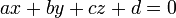we will use the three vertice points, say p1, p2 and p3. From these 3 points, we calculate the normal n as: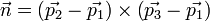then, the plane equation (hence the coefficients a,b,c,d) can be derived by comparing the following equation: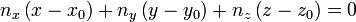Here, (x0,y0,z0) are just the coordinates of any of the vertice points. And finally, the distance D between a point p1(x1,y1,z1) and a plane (a,b,c,d) is given by:Since vector operations are involved, I thought it’s better to do this job in Octave and wrote the following script that calculates the coefficients of the facets and then sets down to find out the points whose distances to all the facets are greater than the given cut-off radius: sururi@husniya trunk$ cat octave_find_atoms_within.m
DummyA = 3;
%conf_index=292;

% Call from the command line like :
%   octave -q --eval "Rcut=1.65;conf_index=293;path='$(pwd)';" octave_find_atoms_within.m % hence specifying the conf_index at the command line. % Emre S. Tasci <e.tasci@tudelft.nl> * % % From the positions and vertices file, calculates the plane % equations (stored in "facet_coefficients") and then % filters the atoms with respect to their distances to these % facets. Writes the output to % "hull_calcs/xxxx_insiders.txt" file % 03/09/09 */ conf_index_name = num2str(sprintf("%04d",conf_index));; %clock clear facet_coefficients; facet_coefficients = []; global p v facet_coefficients fp = fopen(strcat(path,"/hull_calcs/",conf_index_name)); k = fscanf(fp,"%d",2); % 1st is dim (=3), 2nd is number of atoms (=2048) p = fscanf(fp,"%f",[k(1),k(2)]); p = p'; fclose(fp); %p = load("/tmp/del/delco"); fp = fopen(strcat(path,"/hull_calcs/",conf_index_name,".hull")); k = fscanf(fp,"%d",1);% number of facets v = fscanf(fp,"%d",[3,k]); v = v'; fclose(fp); %v = load("/tmp/del/delver"); function [a,b,c,d,e] = getPlaneCoefficients(facet_index) global p v % Get the 3 vertices p1 = p(v(facet_index,1)+1,:); % The +1s come from the fact p2 = p(v(facet_index,2)+1,:); % that qhull enumeration begins p3 = p(v(facet_index,3)+1,:); % from 0 while Octave's from 1 %printf("%d: %f %f %f %f %f %f %f %f %f\n",facet_index,p1,p2,p3); % Calculate the normal n = cross((p2-p1),(p3-p1)); % Calculate the coefficients of the plane : % ax + by + cz +d = 0 a = n(1); b = n(2); c = n(3); d = -(dot(n,p1)); e = norm(n); if(e==0) printf("%f\n",p1); printf("%f\n",p2); printf("%f\n",p3); printf("%d\n",facet_index); endif endfunction function dist = distanceToPlane(point_index,facet_index) global p facet_coefficients k = facet_coefficients(facet_index,:); n = [k(1) k(2) k(3)]; dist = abs(dot(n,p(point_index,:)) + k(4)) / k(5); endfunction for i = [1:size(v)(1)] [a,b,c,d,e] = getPlaneCoefficients(i); facet_coefficients = [ facet_coefficients ; [a,b,c,d,e]]; endfor %Rcut = 1.65; % Defined from command line calling % Find the points that are not closer than Rcut inside_atoms = []; for point = [1:size(p)(1)] %for point = [1:100] inside=true; for facet = [1:size(v)(1)] %for facet = [1:10] %printf("%d - %d : %f\n",point,facet,dist); if (distanceToPlane(point,facet)<Rcut) inside = false; break; endif endfor if(inside) inside_atoms = [inside_atoms point-1]; endif endfor fp = fopen(strcat(path,"/hull_calcs/",conf_index_name,"_insiders.txt"),"w"); fprintf(fp,"%d\n",inside_atoms); fclose(fp); %clock  This script is runned within the following php code which then takes the filtered atoms and does the counting using them: <?PHP /* Emre S. Tasci <e.tasci@tudelft.nl> * * * parse_configuration_data.php * * Written in order to parse the configuation files * obtained from J.F. Wax in order to calculate the * Coordination Number distribution. Each configuration * file contain 2048 atoms' coordinates starting at the * 3rd line. There is also a convex hull file generated * using qhull (http://www.qhull.com) that contains the * indexes (starting from 0) of the atoms that form the * vertices of the convex hull. Finally there is the * IP.dat file that identifies each atom (-1 for K; 1 for * Na -- the second column is the relative masses). * * 02/09/09 */ /* # if present, remove the previous run's results file if(file_exists("results.txt")) unlink("results.txt"); */ error_reporting(E_ALL ^ E_NOTICE); if(!file_exists("results.txt")) {$fp = fopen("results.txt","w");
fwrite($fp,"CONF#"."\tNa".implode("\tNa",range(1,20))."\tK".implode("\tK",range(1,20))."\n"); fclose($fp);
}

# Support for command line variable passing:
for($i=1;$i<sizeof($_SERVER["argv"]);$i++)
{
list($var0,$val0) = explode("=",$_SERVER["argv"][$i]);
$_GET[$var0] = $val0; } if($_GET["configuration"])
calculate($_GET["configuration"]); else exit("Please specify a configuration number [php parse_configuration_data.php configuration=xxx]\n"); function calculate($conf_index)
{
# Define the atoms array
$arr_atoms = Array(); # Get the information from the files parse_types($arr_atoms);
$refs = get_vertices($conf_index);
parse_coordinates($arr_atoms,$conf_index);

# Define the Rcut-off values (obtained from partial parid distribution minimums)
$Rcut_arr = Array();$Rscale = 3.828; # To convert reduced distances to Angstrom (if needed)
$Rscale = 1; # To convert reduced distances to Angstrom$Rcut_arr["Na"]["Na"] = 1.38 * $Rscale;$Rcut_arr["Na"]["K"]  = 1.65 * $Rscale;$Rcut_arr["K"]["Na"]  = 1.65 * $Rscale;$Rcut_arr["K"]["K"]   = 1.52 * $Rscale;$Rcut = $Rcut_arr["Na"]["K"]; # Taking the maximum one as the Rcut for safety /* # We have everything we need, so proceed to check # if an atom is at safe distance wrt to the vertices # Define all the ids$arr_test_ids = range(0,2047);
# Subtract the ref atoms' ids
$arr_test_ids = array_diff($arr_test_ids, $refs); sort($arr_test_ids);
//print_r($arr_test_ids);$arr_passed_atom_ids = Array();
# Check each atom against refs
for($id=0,$size=sizeof($arr_test_ids);$id<$size;$id++)
{
//echo $id."\n";$arr_atoms[$arr_test_ids[$id]]["in"] = TRUE;
foreach ($refs as$ref)
{
$distance = distance($arr_atoms[$arr_test_ids[$id]],$arr_atoms[$ref]);
//echo "\t".$ref."\t".$distance."\n";
if($distance <$Rcut)
{
$arr_atoms[$arr_test_ids[$id]]["in"] = FALSE; break; } } if($arr_atoms[$arr_test_ids[$id]]["in"] == TRUE)
$arr_passed_atom_ids[] =$arr_test_ids[$id]; } */ # Run the octave script file to calculate the inside atoms if(!file_exists("hull_calcs/".sprintf("%04d",$conf_index)."_insiders.txt"))
exec("octave -q --eval \"Rcut=".$Rcut.";conf_index=".$conf_index.";path='$(pwd)';\" octave_find_atoms_within.m"); # Read the file generated by Octave$arr_passed_atom_ids = file("hull_calcs/".sprintf("%04d",$conf_index)."_insiders.txt",FILE_IGNORE_NEW_LINES);$arr_test_ids = range(0,2047);
$arr_test_ids = array_diff($arr_test_ids, $refs); sort($arr_test_ids);
for($i=0,$size=sizeof($arr_test_ids);$i<$size;$i++)
$arr_atoms[$arr_test_ids[$i]]["in"]=FALSE; # Begin checking every passed atom foreach($arr_passed_atom_ids as $passed_atom_id) {$arr_atoms[$passed_atom_id]["in"] = TRUE; for($i=0, $size=sizeof($arr_atoms); $i<$size; $i++) {$distance = distance($arr_atoms[$passed_atom_id],$arr_atoms[$i]);
//echo $passed_atom_id."\t---\t".$i."\n";
if($distance <$Rcut_arr[$arr_atoms[$passed_atom_id]["id"]][$arr_atoms[$i]["id"]] && $distance>0.001)$arr_atoms[$passed_atom_id]["neighbour_count"] += 1; } } # Do the binning$CN_Na = Array();
$CN_K = Array(); for($i=0, $size=sizeof($arr_atoms); $i<$size; $i++) { if(array_key_exists("neighbour_count",$arr_atoms[$i])) {${"CN_".$arr_atoms[$i]['id']}[$arr_atoms[$i]["neighbour_count"]] += 1;
}
}

ksort($CN_Na); ksort($CN_K);

//print_r($arr_atoms); //print_r($CN_Na);
//print_r($CN_K); # Report the results$filename = "results/".sprintf("%04d",$conf_index)."_results.txt";$fp = fopen($filename,"w"); fwrite($fp, "### Atoms array ###\n");
fwrite($fp,var_export($arr_atoms,TRUE)."\n");
fwrite($fp, "\n### CN Distribution for Na ###\n"); fwrite($fp,var_export($CN_Na,TRUE)."\n"); fwrite($fp, "\n### CN Distribution for K ###\n");
fwrite($fp,var_export($CN_K,TRUE)."\n");

# Percentage calculation:
$sum_Na = array_sum($CN_Na);
$sum_K = array_sum($CN_K);
foreach($CN_Na as$key=>$value)$CN_Na[$key] =$value * 100 / $sum_Na; foreach($CN_K  as $key=>$value)
$CN_K[$key]  = $value * 100 /$sum_K;
//print_r($CN_Na); //print_r($CN_K);
fwrite($fp, "\n### CN Distribution (Percentage) for Na ###\n"); fwrite($fp,var_export($CN_Na,TRUE)."\n"); fwrite($fp, "\n### CN Distribution (Percentage) for K ###\n");
fwrite($fp,var_export($CN_K,TRUE)."\n");
fclose($fp); # Write the summary to the results file$fp = fopen("results.txt","a");
for($i=1;$i<=20;$i++) { if(!array_key_exists($i,$CN_Na))$CN_Na[$i] = 0; if(!array_key_exists($i,$CN_K))$CN_K[$i] = 0; } ksort($CN_Na);
ksort($CN_K); fwrite($fp,sprintf("%04d",$conf_index)."\t".implode("\t",$CN_Na)."\t".implode("\t",$CN_K)."\n"); fclose($fp);

}

function parse_types(&$arr_atoms) { # Parse the types$i = 0;
$fp = fopen("IP.DAT", "r"); while(!feof($fp))
{
$line = fgets($fp,4096);
$auxarr = explode(" ",$line);
if($auxarr==-1)$arr_atoms[$i]["id"] = "Na"; else$arr_atoms[$i]["id"] = "K";$i++;
}
fclose($fp); array_pop($arr_atoms);
return 0;
}

function get_vertices($conf_index) {$arr_refs = Array();

# Get the ids of the vertices of the convex hull
$filename = "hull_calcs/".sprintf("%04d",$conf_index).".hull";
$fp = fopen($filename, "r");

# Bypass the first line
$line = fgets($fp,4096);

while(!feof($fp)) {$line = fgets($fp,4096);$auxarr = explode(" ",$line); array_pop($auxarr);
$arr_refs = array_merge($arr_refs, $auxarr); } fclose($fp);
// $arr_refs = array_unique($arr_refs); # This doesn't lose the keys
$arr_refs = array_keys(array_count_values($arr_refs)); # But this does.
return $arr_refs; } function parse_coordinates(&$arr_atoms, $conf_index) { # Parse the coordinates$i = 0;
$filename = "hull_calcs/".sprintf("%04d",$conf_index);
$fp = fopen($filename, "r");

# Bypass the first two lines
$line = fgets($fp,4096);
$line = fgets($fp,4096);

while(!feof($fp)) {$line = fgets($fp,4096);$arr_atoms[$i]["coords"] = explode(" ",$line);
array_shift($arr_atoms[$i]["coords"]);
$i++; } fclose($fp);
array_pop($arr_atoms); return 0; } function distance(&$atom1,&$atom2) { # Calculate the distance between the two atoms$x1 = $atom1["coords"];$x2 = $atom2["coords"];$y1 = $atom1["coords"];$y2 = $atom2["coords"];$z1 = $atom1["coords"];$z2 = $atom2["coords"]; return sqrt(pow($x1-$x2,2) + pow($y1-$y2,2) + pow($z1-$z2,2)); } ?>  The code above generates a “results/xxxx_results.txt” file containing all the information obtained in arrays format and also appends to “results.txt” file the relevant configuration file’s CN distribution summary. The systems can be visualized using the output generated by the following plotter.php script: <?PHP /* Emre S. Tasci <e.tasci@tudelft.nl> * * Parses the results/xxxx_results.txt file and generates * an XYZ file such that the atoms are labeled as the * vertice atoms, close-vertice atoms and inside atoms.. * 02/09/09 */ # Support for command line variable passing: for($i=1;$i<sizeof($_SERVER["argv"]);$i++) { list($var0,$val0) = explode("=",$_SERVER["argv"][$i]);$_GET[$var0] =$val0;
}

if($_GET["configuration"])$conf_index = $_GET["configuration"]; else exit("Please specify a configuration number [php plotter.php configuration=xxx]\n");$filename = sprintf("%04d",$conf_index); # Get the atom array from the results file. Since results file # contains other arrays as well, we slice the file using sed for the # relevant part$last_arr_line = exec('grep "### CN Distribution" results/'.$filename.'_results.txt -n -m1|sed "s:^$$[0-9]\+$$.*:\1:gi"'); exec('sed -n "2,'.($last_arr_line-1).'p" results/'.$filename.'_results.txt > system_tmp_array_dump_file');$str=file_get_contents('system_tmp_array_dump_file');
$atom_arr=eval('return '.$str.';');

# Now that we have the coordinates, we itarate over the atoms to check
# the characteristic and designate them in the XYZ file.
$fp = fopen("system_".$filename.".xyz","w");
fwrite($fp,sizeof($atom_arr)."\n");
fwrite($fp,"Generated by plotter.php\n"); for($i=0, $size=sizeof($atom_arr);$i<$size;$i++) { if(!array_key_exists("in",$atom_arr[$i]))$atomlabel = "Mg";#Vertices
elseif($atom_arr[$i]["in"]==TRUE)
$atomlabel =$atom_arr[$i]["id"];#Inside atoms else$atomlabel = "O";#Close-vertice atoms
fwrite($fp,$atomlabel."\t".implode("\t",$atom_arr[$i]["coords"]));
}
fclose($fp); ?>  which generates a “system_xxxx.xyz” file, ready to be viewed in a standard molecule viewing software. It designates the vertice atoms (of the convex hull, remember? 8)as “Mg” and the atoms close to them as “O” for easy-viewing purpose. A sample parsed file looks like this:The filtered case of a sample Na-K system The big orange atoms are the omitted vertice atoms; the small red ones are the atoms critically close to the facets and hence also omitted ones. You can see the purple and yellow atoms which have passed the test sitting happilly inside, with the counting done using them.. 8) ## Update on the things about the scientific me since October.. ### March 23, 2009 Posted by Emre S. Tasci Now that I’ve started to once again updating this scientific blog of mine, let me also talk about the things that occured since the last entry on October (or even going before that). Meeting with Dr. Villars The biggest event that occured to me was meeting with Dr. Pierre Villars, the person behind the Pauling File database which I have benefitted and still benefitting from. When I had registered to the “MITS 2008 – International Symposium on Materials Database”, an event hosted by the NIMS Materials Database, I had no idea that Dr. Villars would also be attending to the event, so it was a real surprise learning afterwards that I’d have a chance to meet him in person.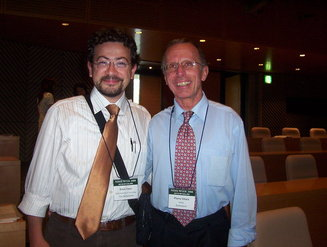You can read the sheer happiness from my face on the occasion of meeting Dr. Villars Dr. Villars is a very kind and a cheerful person, I had a greatest time knowing him and afterwards I had the privilege of visiting him in his home office in the lovely town of Vitznau, Switzerland where I enjoyed the excellent hospitality of the Villars family. Dr. Villars helped me generously in providing the data in my research project for finding transformation toughening materials through datamining which I’m indebted for. The MITS 2008 – Int’l Symposium on Materials Database I was frequently using the databases on the NIMS’ website and had the honour to participate in the last year’s event together with my dear supervisor Dr. Marcel Sluiter. On that occasion, I presented two talks – a materials based one, and another about database query&retrieval methods- titled “Inference from Various Material Properties Using Mutual Information and Clustering Methods” and “Proposal of the XML Specification as a Standard for Materials Database Query and Result Retrieval”, respectively. I have an ever growing interest on the Japanese Culture since I was a youngster and due to this interest, my expectations from this trip to Japan was high enough for me to be afraid of the fact that I may have disappointed since it was next-to-impossible for anything to fulfill the expectations I had built upon. But, amazingly, I was proved false: the experience I had in Japan were far more superior to anything I had anticipated. The hospitality of the organizing commitee was incredible: everything had been taken care of and everyone was as kind as one could be. I had the chance to meet Dr. Villars as I’ve already wrote about above, and also Dr. Xu, Dr. Vanderah, Dr. Dayal and Dr. Sims and had a great time. It was also due to this meeting, I met a dear friend.Ongoing Projects During my time here, I still don’t have anything published and that’s the number one issue that’s bothering me. Apart from my research on the transformation toughening materials, I worked for a time on a question regarding to a related phenomenon, namely about the martensitic transformation mechanism on some transition-metal alloy types. We were able to come up with a nice equation and adequate coefficients that caught the calculation results but couldn’t manage to transfer it to -so to say- a nicely put theory and that doomed the research to being an “ad-hoc” approach. I’ll be returning to it at the first chance I get, so currently, it’s been put on ice (and now remembering how, in the heat of the research I was afraid that someone else would come up with the formula and it would be too late for me.. sad, so sad..). One other (side) project was related to approximate a specific amorphous state in regards with some basis structures obtained using datamining. This project was really exciting and fun to work on because I got to use different methods together: first some datamining, then DFT calculations on the obtained structures and finally thermodynamics to extrapolate to higher temperatures. We’re at the stage of revising the draft at the moment (gratefully!). Life in the Netherlands & at the TUDelft It has been almost one and a half year since I started my postdoc here in TUDelft. When we first came here, my daughter was still hadn’t started speaking (yet she had been practicing walking) and now she approximately spent the same amount of time in the Netherlands as she had spent in Turkey (and thankfully, she can speak and also running to everywhere! 8). When I had come here, I was a nano-physicist, experienced in MD simulations and -independently- a coder when the need arouse. Now, I’m more of a materials guy and coding for the purpose.. 8) I enjoy the wide variety of the jobs & disciplines people around me are working on and definitely benefitting from the works of my friends in our group. I couldn’t ask for more, really. (OK, maybe I’d agree if somebody would come up with an idea for less windy and more sunny weather here in Delft. That’s the one thing I couldn’t still get used to. Delft is a marvelous city with bikes and kind people everywhere, canals full of lillies in the spring, historical buildings everywhere, Amsterdam, Den Haag and Rotterdam just a couple of train stations away and if you wish, Antwerpen, Brussels and Brugges (Belgium) is your next-door neighbour but this dark and closed weather can sometimes be a little mood lowering.) TUDelft is really a wonderful place to be with all the resources and connections that makes you feel you can learn anything and meet anyone that is relevant to the work you’re doing without worrying about the cost. And you’re being kept informed about the events taking place about you through the frequent meetings in your group, in your section, in your department, in your field. I’ve learned a lot and I’m really happy I was accepted here. (And just when you think I was out of photos!.. 8)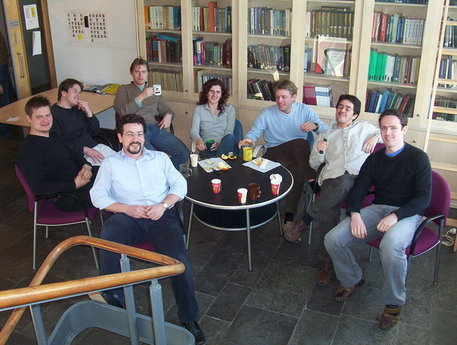So that’s about the things I’ve enjoyed experiencing and living through.. And I promise, I’ll be posting more regularly and frequently from now on.. Yours the chatty. ## Two bash scripts for VASP ### October 20, 2008 Posted by Emre S. Tasci It’s of great importance to have sufficient number of k-points when doing DFT calculations. The most practical (but CPU time costly) way is to check energies for different number of k-points until some kind of "convergence" is reached. Here are the two scripts I’ve recently written for VASP to check for suitable k-point values. The first code runs the calculation with increasing k-points (I have a cubic cell, so using same number of k-points for the 3 lattice axes). Before running this code, make sure that the essential files (KPOINTS POSCAR POTCAR INCAR) are in the directory, also copy your KPOINTS file to a file named "KP" which has KPOINTS mesh defined as 2x2x2: sururi@husniya Al3Li$ cat KP
Automatic
0
Gamma
2 2 2
0 0 0


The following script will then run the calculations, incrementing the number of K-Points by 1 per each axis. It will store the output (and the 4 input files) in archive files in the format k{%03d}.tar.bz2 where the value in the curly brackets is actually the number of k-points per axis. As you can easily see, it runs from k= 1x1x1 (silly but since this is tutorial) to k=13x13x13.

#!/bin/bash

# A script file to run the system for various k-points in order to find the optimal setting
# Emre S. Tasci, 20/10/2008

for (( i = 1; i <= 13; i++ ))
do
date
echo $i # change the KPOINTS (KP is a copy of the KPOINTS with k=2: cat KP|sed s/"2 2 2"/"$i $i$i"/ > KPOINTS

# run the vasp
vasp > vasp.out

# archive the existing files (except the archive files):
tar -cvjf $(printf "k%02d.tar.bz2"$i) $(ls |grep -v "$$.bz2$$\|$$runrunrun.sh$$\|$$KP$$\|$$work$$") # remove the output files: rm$(ls *|grep -v "$$.bz2$$\|$$KPOINTS$$\|$$POSCAR$$\|$$POTCAR$$\|$$INCAR$$\|$$runrunrun.sh$$\|$$KP$$") -f
date
echo "=================================================="
done


After it ends, you should see something like

-rw-r----- 1 sururi users 299K 2008-10-20 10:14 POTCAR
-rw-r--r-- 1 sururi users  283 2008-10-20 10:14 POSCAR
-rw-r--r-- 1 sururi users  604 2008-10-20 10:14 INCAR
-rw-r--r-- 1 sururi users   31 2008-10-20 11:06 KP
-rw-r--r-- 1 sururi users   34 2008-10-20 14:33 KPOINTS
-rw-r--r-- 1 sururi users 195K 2008-10-20 11:08 k01.tar.bz2
-rw-r--r-- 1 sururi users 196K 2008-10-20 11:08 k02.tar.bz2
-rw-r--r-- 1 sururi users 193K 2008-10-20 11:08 k03.tar.bz2
-rw-r--r-- 1 sururi users 195K 2008-10-20 11:08 k04.tar.bz2
-rw-r--r-- 1 sururi users 195K 2008-10-20 11:08 k05.tar.bz2
-rw-r--r-- 1 sururi users 197K 2008-10-20 11:09 k06.tar.bz2
-rw-r--r-- 1 sururi users 197K 2008-10-20 11:09 k07.tar.bz2
-rw-r--r-- 1 sururi users 200K 2008-10-20 11:10 k08.tar.bz2
-rw-r--r-- 1 sururi users 201K 2008-10-20 11:10 k09.tar.bz2
-rw-r--r-- 1 sururi users 205K 2008-10-20 11:11 k10.tar.bz2
-rw-r--r-- 1 sururi users 205K 2008-10-20 11:12 k11.tar.bz2
-rw-r--r-- 1 sururi users 211K 2008-10-20 11:13 k12.tar.bz2
-rw-r--r-- 1 sururi users 211K 2008-10-20 11:14 k13.tar.bz2
-rwxr--r-- 1 sururi users  732 2008-10-20 14:02 runrunrun.sh

Now, it’s time for the second script. Create a new directory (say “work”) and then type the following into a script file (don’t forget to set it as executable (or you can also always “sh ” 😉 ) )

#!/bin/bash

# Script name: energy_extract_from_OUTCAR.sh
# Emre S. Tasci, 20/10/2008

# Reads the OUTCAR files from the archive files in the parent directory
# Stores the energies in corresponding ENE files.

# (Assuming filenames are given like "k05.tar.bz2")

k=00

for i in ../k*.bz2
do
kpre=$k enepre="0.00000000" # Extract OUTCAR tar -xjf$i OUTCAR

# Parse file counter from filename
k=$(echo$(basename $i)|sed "s:k$$[0-9]*$$.*:\1:") # write the energies calculated in this run cat OUTCAR | grep "energy without"|awk '{print$8}' > $(printf "%s_ENE"$k)

#calculate the energy difference between this and the last run
if [ -e "$(printf "%s_ENE"$kpre)" ]
then
enepre=$(tail -n1$(printf "%s_ENE" $kpre)); fi enethis=$(tail -n1 $(printf "%s_ENE"$k));

# Using : awk '{ print ($1 >= 0) ?$1 : 0 - $1}' : for absolute value echo -e$(printf "%s_ENE" $kpre) " :\t"$enepre "\t" $(printf "%s_ENE"$k) ":\t" $enethis "\t"$(echo $(awk "BEGIN { print$enepre - $enethis}") | awk '{ print ($1 >= 0) ? $1 : 0 -$1}')

rm -f OUTCAR
done;


when runned, this script will produce an output similar to the following:

sururi@husniya work $./energy_extract_from_OUTCAR.sh 00_ENE : 0.00000000 01_ENE : -6.63108952 6.63109 01_ENE : -6.63108952 02_ENE : -11.59096452 4.95988 02_ENE : -11.59096452 03_ENE : -12.96519853 1.37423 03_ENE : -12.96519853 04_ENE : -13.20466179 0.239463 04_ENE : -13.20466179 05_ENE : -13.26411934 0.0594576 05_ENE : -13.26411934 06_ENE : -13.26528991 0.00117057 06_ENE : -13.26528991 07_ENE : -13.40540825 0.140118 07_ENE : -13.40540825 08_ENE : -13.35505746 0.0503508 08_ENE : -13.35505746 09_ENE : -13.38130280 0.0262453 09_ENE : -13.38130280 10_ENE : -13.36356457 0.0177382 10_ENE : -13.36356457 11_ENE : -13.37065368 0.00708911 11_ENE : -13.37065368 12_ENE : -13.37249683 0.00184315 12_ENE : -13.37249683 13_ENE : -13.38342842 0.0109316  Which shows the final energies of the sequential runs as well as the difference of them. You can easily plot the energies in the gnuplot via as an example “plot “12_ENE”“. You can also plot the evolution of the energy difference with help from awk. To do this, make sure you first pipe the output of the script to a file (say “energies.txt”): ./energy_extract_from_OUTCAR.sh > energies.txt And then, obtaining the last column via awk cat energies.txt |awk '{print$7}' > enediff.txt

Now you can also easily plot the difference file.

Hope this scripts will be of any help.

### August 7, 2008 Posted by Emre S. Tasci

Still saddened by the loss of Randy Pausch whom I knew about from his famous "Last Lecture", while working on the Miedema’s Model, I came across this article by him: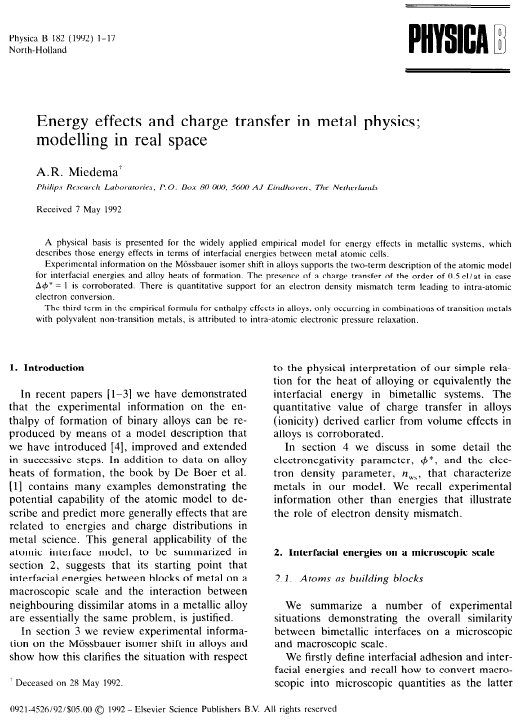Dr. Villars had already mentioned Miedema’s passing at a young age but still it was really tragic to find such a sad detail on a scientific article.

I checked the web for a Miedema biography but couldn’t find any. The only information I could find was from H. Bakker’s book which I’m quoting the preface below:

PREFACE

Andries Miedema started developing his rather unconventional model in the sixties as a professor at the University of Amsterdam and continued this work from 1971 at Philips Research Laboratories. At first he encountered scepticism in these environments, where scientists were expecting all solutions from quantum- mechanics. Of course, Miedema did not deny that eventually quantum mechanics could produce (almost) exact solutions of problems in solid-state physics and materials science, but the all-day reality he experienced was, – and still is -, that most practical problems that are encountered by researchers are too complex to allow a solution by application of the Schr6dinger equation. Besides, he believed in simplicity of nature in that sense that elements could be characterised by only a few fundamental quantities, whereby it should be possible to describe alloying behaviour. On the basis of a comparison of his estimates of the heat of formation of intermetallic compounds with ample experimental data, he was gradually able to overcome the scepticism of his colleagues and succeeded in convincing the scientific world that his model made sense. This recognition became distinct by the award of the Hewlett Packard prize in 1980 and the Hume-Rothery decoration in 1981. At that time, Miedema himself and many others were successfully using and extending the model to various questions, of course realising that the numbers obtained by the model have the character of estimates, but estimates that could not be obtained in a different fast way. It opened new perspectives in materials science. Maybe the power of the model is best illustrated by a discussion, the present author had with Jim Davenport at Brookhaven National Laboratory in 1987. Dr Davenport showed him a result that was obtained by a one-month run on a Gray 1 supercomputer. This numerical result turned out to be only a few kilo Joules different from the outcome by Miedema’s model, obtained within a few minutes by use of a pocket calculator. Andries Miedema passed away in 1992 at a much too young age of 59 years. His death came as a bad shock not only for the scientific community in The Netherlands, but for scientists all over the world. However, for his family, friends and all the people, who knew him well, it is at least some consolation that he lives on by his model, which is being used widely and which is now part of many student programmes in physics, chemistry and materials science.

It is the aim of the present review to make the reader familiar with the application of the model, rather than to go in-depth into the details of the underlying concepts. After studying the text, the reader should be able, with the aid of a number of tables from Miedema’s papers and his book, given in the appendix, to make estimates of desired quantities and maybe even to extend the model to problems that, so far, were not handled by others. Beside, the reader should be aware of the fact that not all applications could be given in this review. For obvious reasons only part of all existing applications are reported and the reader will find further results of using the model in Miedema’s and co-worker’s papers, book and in literature.

Dr Hans Bakker is an associate professor at the Van der Waals-Zeeman Institute of the University of Amsterdam. In 1970 Andries Miedema was the second supervisor of his thesis on tracer diffusion. He inspired him to subsequent work on diffusion and defects in VIII-IIIA compounds at a time, when, – as Bakker’s talk was recently introduced at a conference -, these materials were not yet relevant’ as they are now. Was it again a foreseeing view of Andries Miedema? Was it not Miedema’s standpoint that intermetallic compounds would become important in the future?

Rather sceptic about the model as Bakker was at first like his colleagues, his scepticism passed into enthusiasm, when he began using the model with success in many research problems. In 1984 Bakker’s group started as one of the first in the world ball milling experiments. Surprisingly this seemingly crude technique turned out to be able to induce well-defined, non-equilibrium states and transformations in solids. Miedema’s model appeared again to be very helpful in understanding and predicting those phenomena.

Hans Bakker
Mauro Magini

Preface from Enthalpies in Alloys : Miedema’s Semi-Emprical Model
H. Bakker
Trans Tech Publications 1998 USA
ISSN 1422-3597